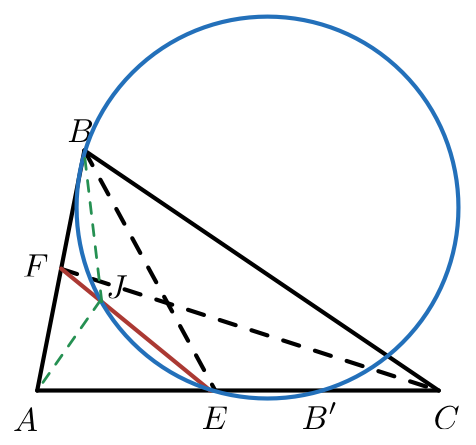# How can you relate these two points?

Geometry Level 5In $\triangle ABC$, $\angle A=80^\circ, \angle B=65^\circ ,\angle C=35^\circ$. The internal angle bisectors of $\angle B,\angle C$ meet their respective opposite sides at $E,F$. Let $B'$ be a point on segment $AC$ such that $AB'=AB$. Let the circumcircle of $BB'E$ intersects $FE$ at $J$.

Find the measure (in degrees) of $\angle AJB$.

×